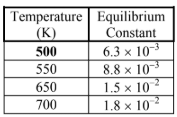# Problem: The table below lists the equilibrium concentrations for H 2(g), I2(g), and HI(g) in the given reaction.H2 (g) + I2 (g) ⇌ 2HI (g)At 500 K, the gases have equilibrium partial pressures of PI2 = 1.00 atm, PH2 = 1.00 atm, and PHI = 0.0795 atm. An addition of 0.100 atm HI(g) is made to this system.Determine the final partial pressures for H2(g), I2(g), and HI(g) when the system returns to equilibrium (i.e., after the addition of 0.100 atm HI(g)). a. PI2 = 1.086 atm, PH2 = 1.086 atm, and PHI = 0.00695 atmb. PI2 = 1.275 atm, PH2 = 1.275 atm, and PHI = 0.0225 atmc. PI2 = 1.621 atm, PH2 = 1.621 atm, and PHI = 0.00731 atmd. PI2 = 1.072 atm, PH2 = 1.072 atm, and PHI = 0.00642 atme. PI2 = 1.372 atm, PH2 = 1.372 atm, and PHI = 0.00614 atm

###### FREE Expert Solution
92% (373 ratings)View Complete Written Solution
###### Problem Details

The table below lists the equilibrium concentrations for H 2(g), I2(g), and HI(g) in the given reaction.

H(g) + I(g) ⇌ 2HI (g)At 500 K, the gases have equilibrium partial pressures of PI2 = 1.00 atm, PH2 = 1.00 atm, and PHI = 0.0795 atm. An addition of 0.100 atm HI(g) is made to this system.

Determine the final partial pressures for H2(g), I2(g), and HI(g) when the system returns to equilibrium (i.e., after the addition of 0.100 atm HI(g)).

a. PI2 = 1.086 atm, PH2 = 1.086 atm, and PHI = 0.00695 atm

b. PI2 = 1.275 atm, PH2 = 1.275 atm, and PHI = 0.0225 atm

c. PI2 = 1.621 atm, PH2 = 1.621 atm, and PHI = 0.00731 atm

d. PI2 = 1.072 atm, PH2 = 1.072 atm, and PHI = 0.00642 atm

e. PI2 = 1.372 atm, PH2 = 1.372 atm, and PHI = 0.00614 atm

Frequently Asked Questions

What scientific concept do you need to know in order to solve this problem?

Our tutors have indicated that to solve this problem you will need to apply the The Reaction Quotient concept. You can view video lessons to learn The Reaction Quotient. Or if you need more The Reaction Quotient practice, you can also practice The Reaction Quotient practice problems.

What is the difficulty of this problem?

Our tutors rated the difficulty ofThe table below lists the equilibrium concentrations for H 2...as high difficulty.